# Wave Optics: Interference and Diffraction

Student Learning Objectives
Lessons / Lecture Notes
Important Equations
Example Problems
Applets and Animations

Student Learning Objectives

• To introduce and use the wave model of light.
• To recognize the experimental evidence for the wave nature of light.
• To understand how and why interference of light occurs.
• To understand and calculate the interference patterns of double slits and diffraction gratings.
• To study the inevitable spreading of waves due to diffraction.
• To understand how the index of refraction affects light waves in a medium.
• To understand the conditions for constructive and destructive interference in thin films.
• To understand how light diffracts through single slits and circular apertures.
• To understand how diffraction limits the resolution of optical instruments.

Lessons / Lecture Notes

The Physics Classroom (conceptual)

PY106 Notes from Boston University (algebra-based):

Physics 2C notes from Dr. Bobby W.S. Lau (algebra-based)

HyperPhysics (calculus-based)

Physics 4C notes from Dr. Bobby W.S. Lau (calculus-based)

Important Equations (for algebra-based Physics)

Example Problems

Example Problems for algebra-based physics (from Physics 8th Edition by Cutnell and Johnson):

Example Problems (Interference)
Solutions to Example Problems (Interference)

Example Problems for calculus-based physics (from Fundamentals of Physics 9th Edition by Halliday, Resnick, and Walker):

Example Problems (Interference) | Example Problems (Diffraction)
Solutions to Example Problems (Interference) | Solutions to Example Problems (Diffraction)

Applets and Animations
 Huygen's PrincipleAnimation shows a plane wave front spreading through a small opening. The slit dimensions are considered to be very small compared to the wavelength of the waves. Huygens-Fresnel Principle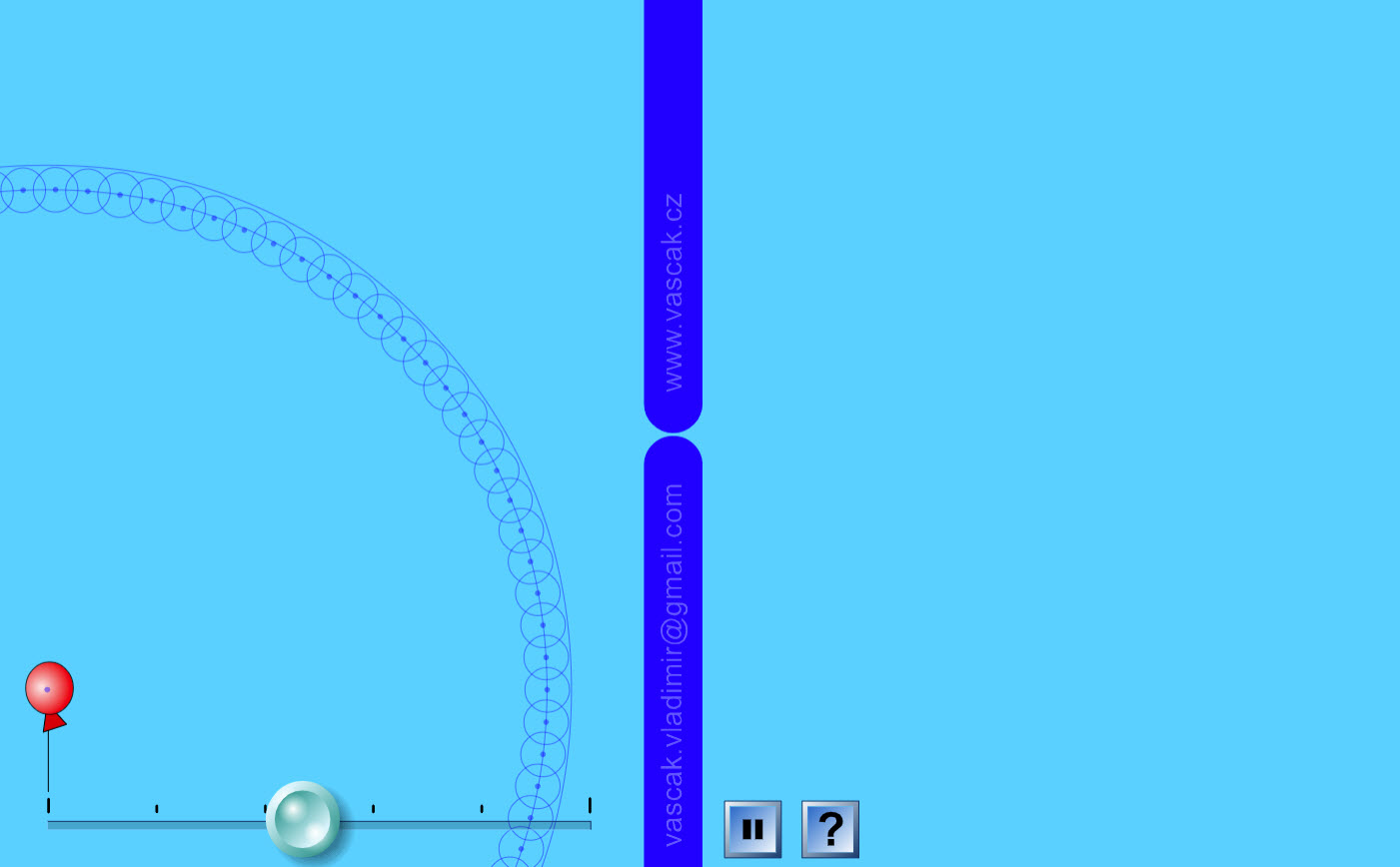A nice animation showing Huygen's Principle. Diffraction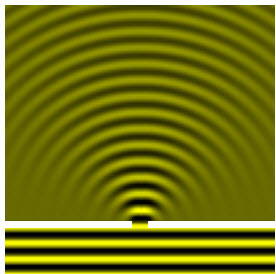Animation showing the diffraction of waves through an opening. Wave Interference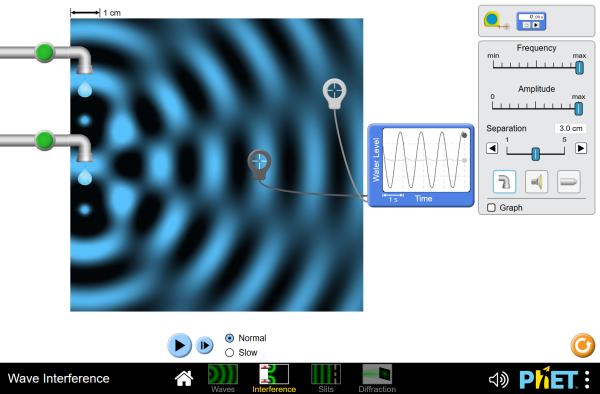Make waves with a dripping faucet, audio speaker, or laser! Add a second source to create an interference pattern. Put up a barrier to explore single-slit diffraction and double-slit interference. Experiment with diffraction through elliptical, rectangular, or irregular apertures. Interference of Water Waves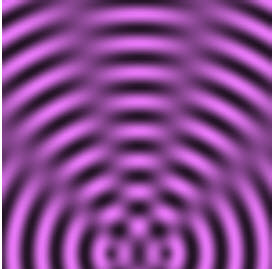An nice animation showing the interference of water waves. The user can adjust the wavelength, source separation, and the phase difference between the sources. Two-Source Interference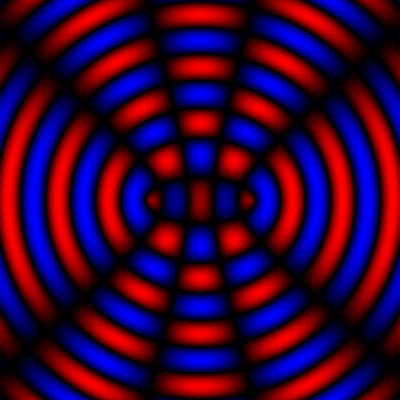This simulation demonstrates interference of waves from two identical sources that are separated by a variable distance. The wave crests are shown in red and the troughs in blue, with black indicating a local wave amplitude of zero. (Note that the entire image updates instantly when you move the slider, because it is drawn using a formula that assumes fixed separation, rather than from a simulation of the time-dependent response of the wave medium. Note also that the wave amplitude does not decrease with the distance from the source, as it would for physical waves.) Two-Source Interference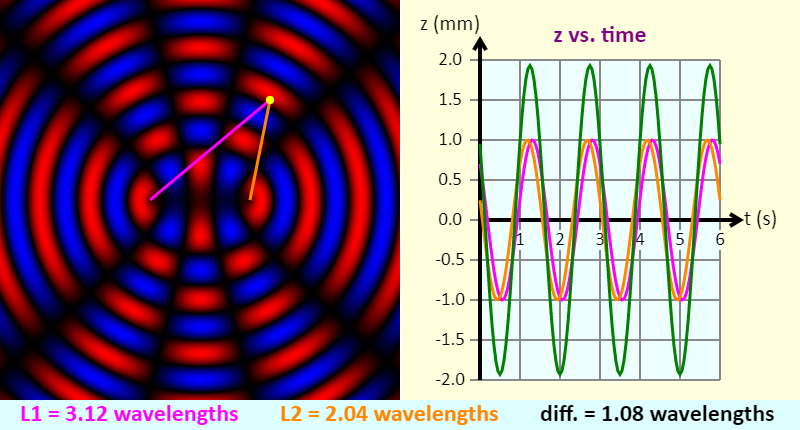This simulation demonstrates interference of waves from two identical sources that are separated by a variable distance. The wave crests are shown in red and the troughs in blue, with black indicating a local wave amplitude of zero. Alternatively, you can switch to black-and-white, using the Grayscale button - in that case both the peaks and troughs are white. (Note that the entire image updates instantly when you move the slider, because it is drawn using a formula that assumes fixed separation, rather than from a simulation of the time-dependent response of the wave medium. Note also that the wave amplitude does not decrease with the distance from the source, as it would for physical waves.) You can click-and-drag on the yellow point to change its position. When is there constructive interference at the yellow point, and when is there destructive interference at that point? The graph shows the displacement at the yellow point because of the left source (the pink graph), the right source (the orange graph), and the net displacement (in green). Double Slit Wave Interference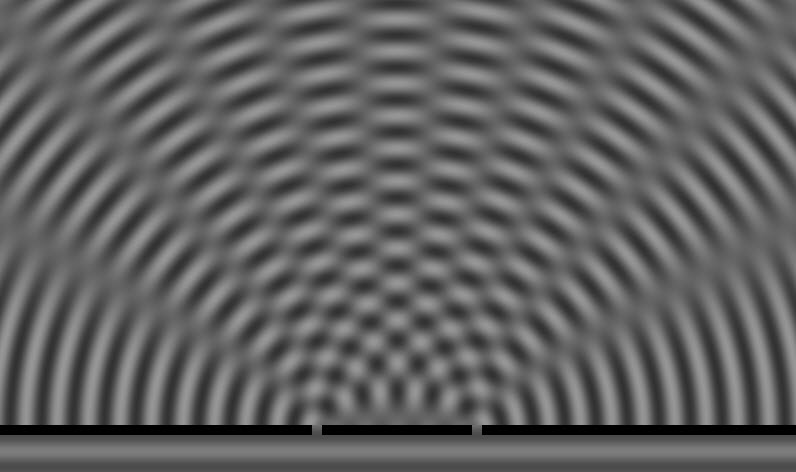This applet shows the double-slit interference pattern from water waves. Double-Slit Experiment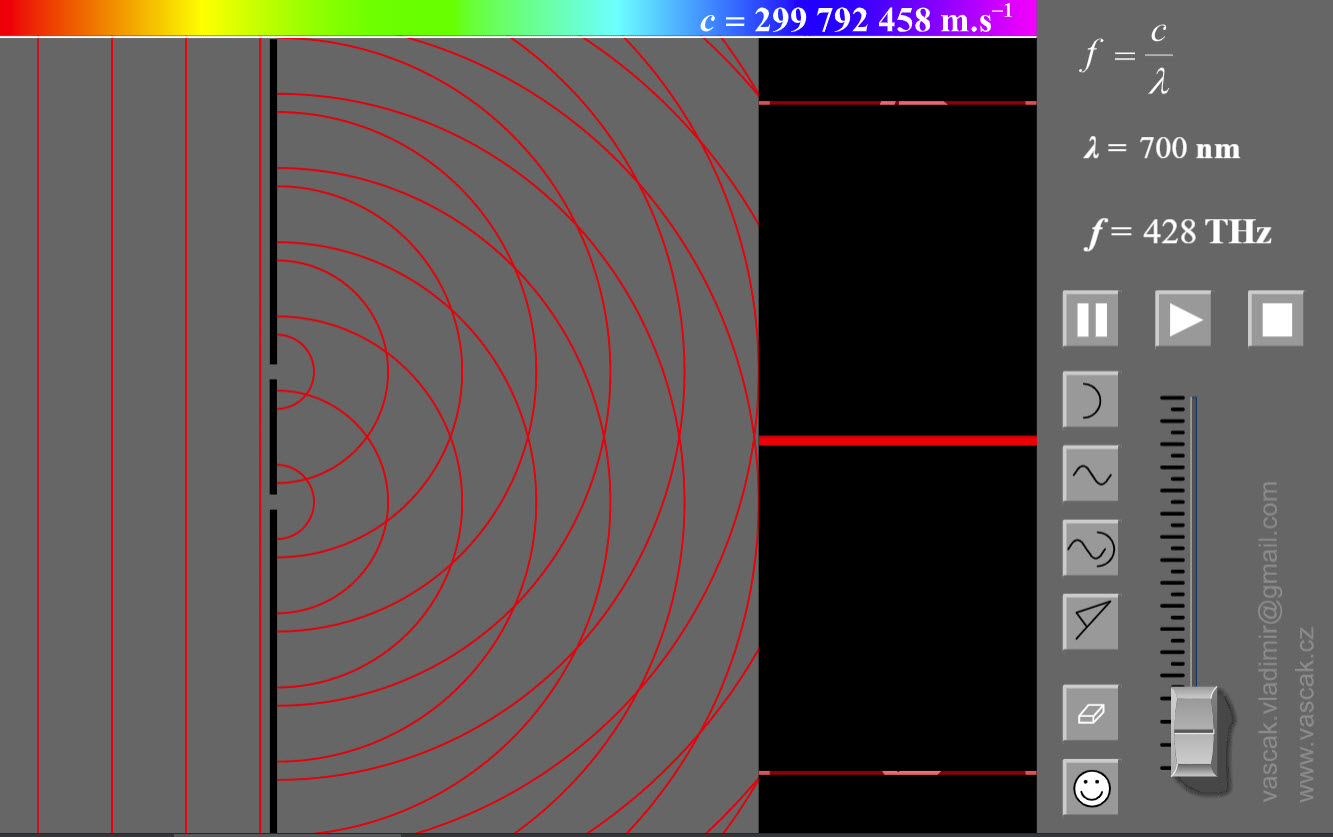This excellent applet shows double-slit interference of light waves. There are numerous options the user can change. Interference of Light at a Double Slit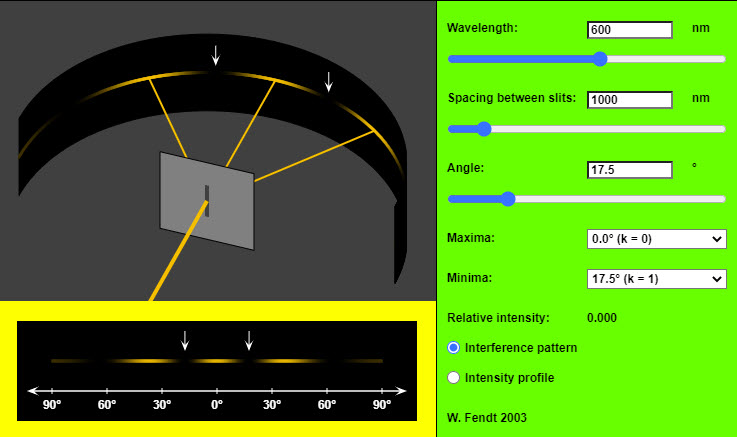A very nice applet showing interference of light at a double slit. Young's Interference Experiment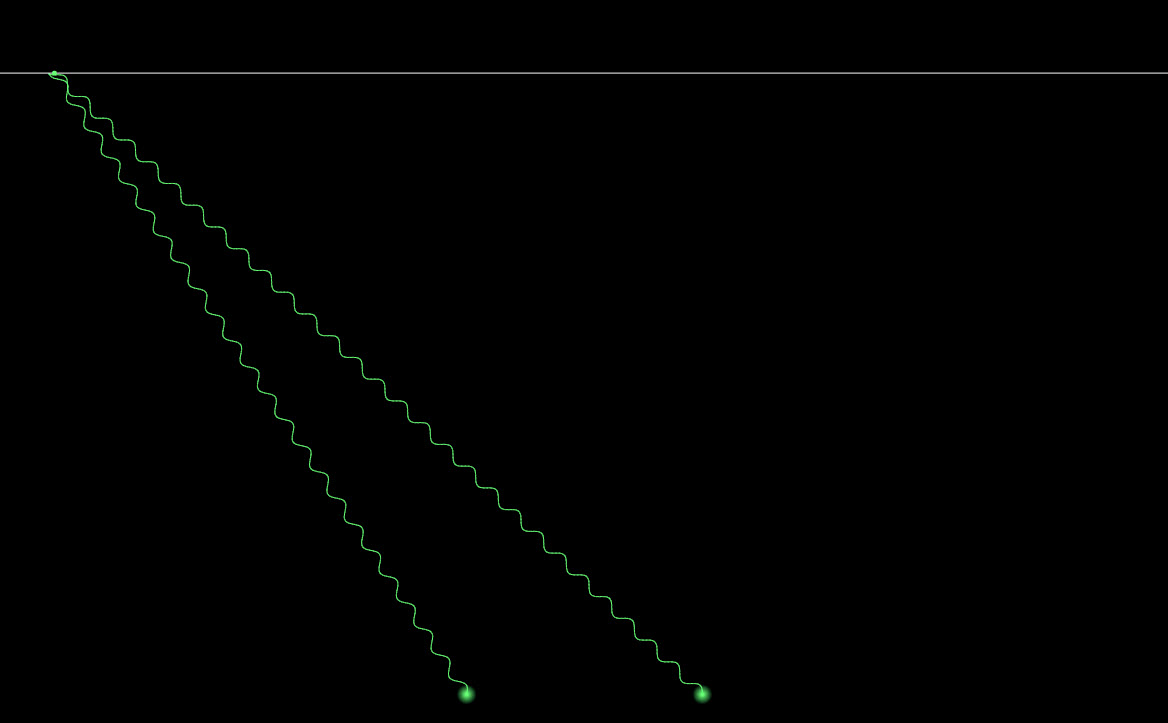A simple animation showing Young's interference experiment. Young's Double SlitChoose between experiment and results to view either from the menu. Measurements are not to scale. Dragging changes the view point in experiment option. Double click/tap resets to original view. Intensity on the screen due to each slit is assumed to be uniform. Diffraction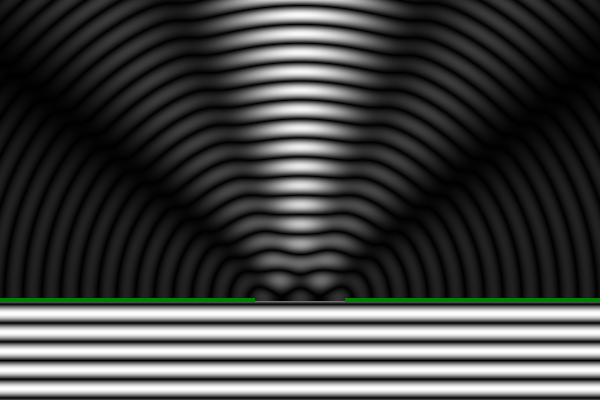This simulation demonstrates the diffraction of waves by a single slit. The width of the opening is controlled by the slider. Initially, the waves are shown in black and white (grayscale), with both the peaks and troughs being white. Black indicates zero amplitude. Alternatively, you can switch to a red and blue color scheme, in which the wave crests are shown in red and the troughs in blue, with black still indicating zero amplitude. Note that the wave amplitude does not decrease with the distance from the source, as it would for physical waves. Diffraction of Light by a Single Slit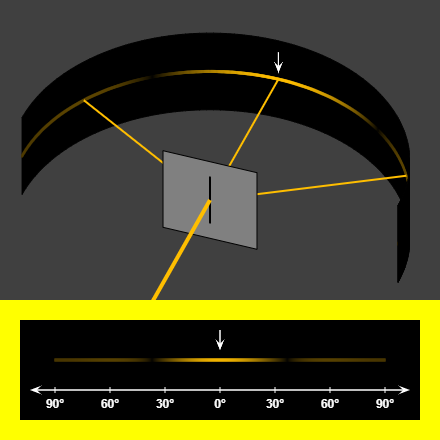A very nice applet showing diffraction of light by a single slit. Single Slit Diffraction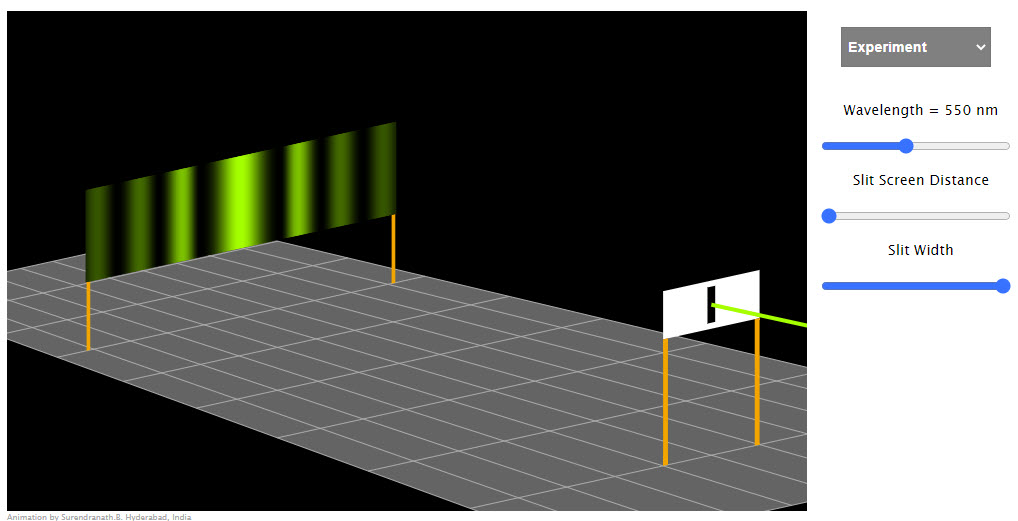Choose between experiment and results to view either from the menu. When results are displayed, dragging below the graph area shows the alignment of individual phasors (white) and the resultant phasor (yellow). The intensity in the sidebands is exaggerated to make them visible. You can increase, decrease the scale on the y axis by clicking/touching in the right/left halves of the graph area. Double SlitAn outstanding applet showing double-slit diffraction. Diffraction GratingThis is a simulation of a what light does when it encounters a diffraction grating. Use at least one of the checkboxes to turn on a beam of light. When the light encounters the diffraction grating (a comb-like structure with a large number of equally spaced openings), the light is diffracted, because of constructive interference, at particular angles. Compared to a double-slit, the lines produced by the diffraction grating are very sharp (narrow) and bright. A close-up of the center of the grating can be seen at the bottom right. You can use the slider to control the grating spacing, which is the distance between neighboring openings in the grating. In the simulation, red light has a wavelength of 650 nm, green light has a wavelength of 550 nm, and blue light has a wavelength of 450 nm. Thin-Film Interference SimulationWith this simulation, you can explore thin-film interference. When light traveling in one medium is incident on a thin film of material that is in contact with another medium, some light reflects off the front surface of the film, and some light goes through the film, reflects off the back surface of the film, and emerges back into the original medium. These two reflected waves then interfere with one another. The interference can be constructive, destructive, or something in between, depending on the thickness of the film. Newton's Rings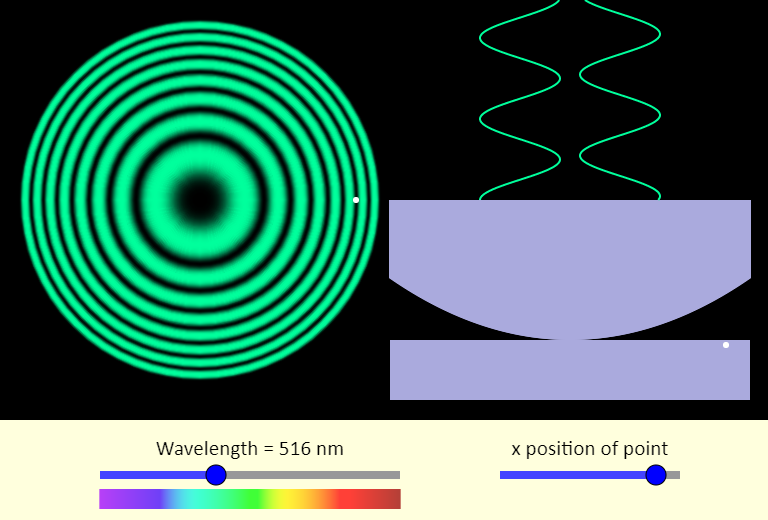In Newton's rings, a curved watch glass sits on top of a flat piece of glass. A thin film of air is in between the two - the thin film has a thickness that is zero where the two pieces of glass touch, and gradually increases as you move away from that point. Light of a single wavelength shines down from above. Light reflecting from the top and bottom surfaces of the thin film interfere, leading to a bulls-eye pattern, as we see on the left (the overhead view). On the right is the side view. Note that the thickness of the thin film is not shown to the same scale as the wavelength of the two reflected waves - those waves show the interference, which can be either constructive or destructive. Use the sliders to change the wavelength (how does that affect the pattern?), and the point where we consider the interference in the film.